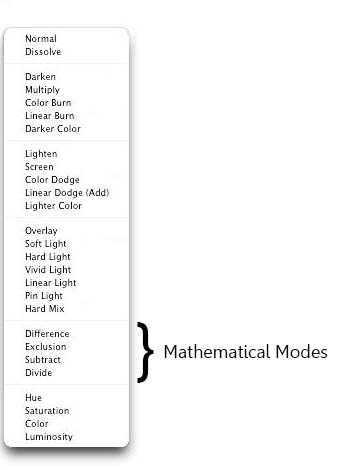# Photoshop Basics – Mathematical Blending Modes

So far we have covered the top four categories of the blend modes. We will now move on to the 5th category, known as Mathematical blending modes.The four blend modes in this category are actually known by other names as well. For example, the first two blend modes, Difference and Exclusion are also known as comparative as well as Inversion modes while Subtract and Divide are also known as cancellation modes. I on the other hand simply like to call them the mathematical modes. Now we have already learnt in our previous tutorials that there is an actual math involved with the way each of the blend modes work. We did not actually get into the math earlier and tried to understand the effect of the blend mode without getting into any sort of calculation but unfortunately, with our mathematical category, we will have to dig into math a little. Good part however is that these are also one of the least used blend modes of Photoshop so we do not need to spend much time here. I will cover all these four modes in the same tutorial rather than explaining each of them separately.

Let us first look at the official definition of these modes, per Photoshop help site.

1. Difference : Looks at the color information in each channel and subtracts either the blend color from the base color or the base color from the blend color, depending on which has the greater brightness value. Blending with white inverts the base color values; blending with black produces no change.

2. Exclusion : Creates an effect similar to but lower in contrast than the Difference mode. Blending with white inverts the base color values. Blending with black produces no change.

3. Subtract : Looks at the color information in each channel and subtracts the blend color from the base color. In 8- and 16-bit images, any resulting negative values are clipped to zero.

4. Divide : Looks at the color information in each channel and divides the blend color from the base color.”

Simply put, every color in Photoshop has a numeric value to it. For example, for a pure red color, the values will be: red =255 green=0 blue=0. See the screenshot below for reference.

As we move to a different color, the RGB fields will display the numeric value of that color. Keeping that in mind, this is what happens when you apply any of the mathematical blend modes.

1. Difference blending mode highlights the differences between the blend layer and the base layer. The more technical explanation is that the blend color is subtracted from the base color–or vice-versa, depending on the brightness–and the result is the difference between them. When white is the blend color, the base image is inverted. When black is the blend color, there is no change.

2. The Exclusion blending mode works very much like Difference but the contrast is lower. When white is the blend color, the base image is inverted. When black is the blend color, there is no change.

3. Subtract : Looks at the color information in each channel and subtracts the blend color from the base color. In 8- and 16-bit images, any resulting negative values are clipped to zero.

4. Divide : Looks at the color information in each channel and divides the blend color from the base color.”

All the four mathematical blend modes are used mostly for image alignment and special effects. For your day to day editing, it would be very rare that you will end up using the mathematical modes.

#### 1 commentApril 16, 2020 - 5:06 pm

Please add some examples and calculations also. It was really a nice article.